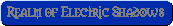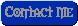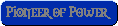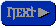# Mass As An Electromagnetic Effect

T.E. Bearden
Association of Distinguished American Scientists
2311 Big Cove Road, Huntsville, Alabama 35801Fax to David Jonsson, Upsala, Sweden (internet: david@ibg.uu.se)
June 1, 1995

Dear David:

I will prepare a diskette for you with the material (the fax) on it. Later, I will follow with a diskette of the technical paper, which will have some dozen or so drawings as well.

In reference to mass as an electromagnetic effect: the equation E=mc2 can be taken to state the "EM energy trapped in mass" is equal to mc2; since energy can be transformed from one form to another, we are free to pick EM energy. The problem immediately rises on the difference between "energy" and "energy density flow". All EM energy must be in motion at the speed of light, by Einstein's postulate, with respect to any observer. So how can it be seen by the external observer as "sitting still" so as to comprise mass? The answer can be found in E.T. Whittaker, "On the Partial Differential Equations of Mathematical Physics", Mathematische Annalen, Vol. 57, 1903, p.333-355. Whittaker mathematically decomposes the scalar potential into a harmonic series of bidirectional EM wave pairs. Each wavepair consists of the wave and its phase conjugate. By the distortion correction theorem of nonlinear optics (which did not exist until the mid-1970s), in each wavepair the wave and its antiwave (phase conjugate replica) must spatially superpose, though antiphased in the time dimension. Therefore each wavepair is a special kind of standing wave; where at each point an E of the wave and -E of the antiwave simultaneously superpose. In the superposition, [E + (-E)] individual vectors do not "cancel" and "cease to exist", just as two elephants straining against each other do not "cancel" and "disappear" just because the translation of the two-elephant system is zero. Each elephant is still in there and, as an individual, he is straining mightily. In the wave/antiwave case, the waves pass through the same space exactly superposed spatially, as seen by the observer. Now to be "seen by the observer", actually a little observation time is required. In other words, what is detected by observation is not E or -E, but Et or -Et. The observation time-differentiates, to get rid of the t. So the "observer" will "see" a zero net E-field, composed of E + (-E) = 0. The scalar field energy densities add at any point, however, while their observed "resultant vector summation" E-field is a zero vector. All the zero vector means is that the system of "seemingly trapped" superposed EM energy at that point is not translating. The energy is not really trapped, in the same way that the water flowing through a steady whirlpool in a river is not static. The whirlpool form (the "collection" form of the water flow) seems static. The flow continues. So it is with so-called "trapped" EM energy.

Thus a pure gravitational standing wave is nothing in the world but an ordinary EM wave plus its superposed true phase conjugate replica wave (its time-reversed wave twin). For this reason, all potentials are gravitational, as is already recognized in general relativity. As you look along the wave/antiwave pair, along any radius from the "transmitter", so to speak, you see a sinusoidal variation of the local trapped EM energy density of the vacuum, as a function of the radius. So you see electromagnetics turned into gravitation.

We now insist on strong phase conjugation. I.e., if the wave is phase conjugated to form a time-reversed replica or antiwave, every photon comprising the wave must also be individually time-reversed, else the antiwave is not a true phase conjugate replica microscopically.

So in the wave/antiwave Whittaker pair comprising the potential, the wavepair is made of photon/antiphoton pairs. These are spin-2 entities, hence they are gravitons. So the electrostatic scalar potential is formed of gravitons, not individual photons. (Cooper pairs, eat your heart out!)

Any electric dipole will separate the two waves comprising the Whittaker pair. That is, one end (the negative end) of the dipole is time-forward. The positive end is time-reversed. So in each wavepair, the two waves "split" due to their difference in the time dimension. The wave interacts with the negative end of the dipole, giving us our familiar EM interaction. The antiwave interacts with the positive (time-reversed) end of the dipole, giving us the familiar "Newtonian third law reaction". In an atom, one can choose an electron in the orbital shells, and a corresponding positive charge in the nucleus, and call that a dipole. The atom then becomes a complex arrangement of dynamic electrical dipoles, for this model. When the wavepair (wave plus its antiwave) comes in, a dipole receives it. The wave interacts with the orbital electron, giving us the familiar "photon" interaction. At the same time, the antiwave is split off and interacts with the other end of the dipole in the nucleus, giving us the familiar nuclear recoil. Since the nucleus is much more massive than the electron, we normally (in antennas and receivers) do not even take it into account.

However, we have (in other places) shown that the wave in the vacuum is never a transverse EM wave anyway. Maxwell simply assumed the transverse string wave. In fact, it is simple to prove that the wave in the vacuum is a longitudinal "sound" type wave. A spinning electron in the free electron gas in a receiving wire antenna, e.g., is longitudinally restrained by the repulsion of myriads of electrons beyond it in the gas. It is also spinning. Consequenly, it must act as a gyro, where its spin axis is longitudinally restrained (it can only "drift" at electron drift velocity down the wire; the disturbing force moves down the wire at lightspeed). Since the spinning electron is a gyro, it will precess when a disturbing force is impressed. Specifically, it will precess at right angles to that disturbing force, by the theory of gyroscopes. So simply see which way the electrons moves, and the disturbing force that came in from the vacuum had to be at right angles to that electron movement. Voila! The electron does indeed move laterally (transversally). Hence the vacuum disturbance is longitudinal. QED.

EM waves in vacuum have always been longitudinal waves. Again, Maxwell assumed the plucked string transverse wave. [Note that the wave in a plucked string is the wave in the string only, it never leaves the string, and it is not the wave that launches into the air]. In deriving this plucked string transverse wave, the old boys assumed infinitely rigid endholders for the taut string. No such thing exists in nature. What really exists is a fairly rigid endholder. However, the body of the instrument produces a wave of equal and opposite energy, but highly damped in magnitude because of the denser medium of the endholders and the instrumental body of the instrument. Nonetheless, an antiwave is produced in the body of the instrument equal in energy to the string wave. This is still missing from the standard sophomore's derivation of the wave equation from the taut string example. The string then imparts its energy density to the air medium, as does the body of the instrument. The two (wave and antiwave) are of equal energy. Now that they are in the same medium, they again become the same magnitude because of their equal energy. Voila! One has two antiphased transverse waves, moving in the same direction, which thus forms a longitudinal wave of compression and rarefaction.

The same thing is true of an EM wave. When the wave we have in our textbooks is created in the electron gas in, say, a long copper wire antenna, an equal-energy but highly damped antiwave is created in the atomic nuclei of the copper wire. The nuclei are positively charged, hence time-reversed. The equal energy antiwave in the atomic nuclei thus is a phase conjugate replica of the wave in the electron gas, and of equal energy through highly damped in magnitude. When the "transverse" EM wave energy is impressed upon the vacuum medium by the oscillating electron gas of the copper wire, and equal energy phase conjugate replica wave is impressed upon the same vacuum medium by the oscillating nuclei. Once in the vacuum medium, the damping is the same for both waves, so they now suddenly assume equal magnitudes. Voila! One has a wavepair, of two transverse waves, one timeforward and the other time-reversed, just as one of the pairs in Whittaker's decomposition of the scalar potential.

In short, we have a longitudinal wave, because at each point in space the wave's Et and the antiwave's corresponding -Et cancel in the 4th dimension. They do not cancel in 3-space, but add. Thus the energies of the wave add at each point. We have already described how this wave is detected and received by an atom in a receiving antenna.

I hope this convinces you that EM and G are already linked closely, and that EM theory is seriously flawed.

We can further prove it this way. A phase conjugate mirror (PCM) material "tricks" the antiphoton into going out of the atom instead of going into the nucleus to interact. It does this, no matter how powerfully pumped. So it follows that a PCM must not exhibit Newtonian third law recoil! And it doesn't. Experimentally, a pumped phase conjugate mirror does not recoil, no matter how powerfully it is pumped! The mechanism to generate Newton's third law is prevented from occuring in the material. The emitted phase conjugate replica wave (the time-reversed wave) contains all the antiphotons that would normally have interacted in the nuclei to produce 3rd law recoil.

Note that a PPCM proves conclusively that it is perfectly possible to directly engineer Newton's third law. I hope you can see that this proves that unidirectional forces can be created, by applying such engineering. It also proves that back emf's etc. can also be engineered.

In short, it proves that overunity electrical machines and antigravity devices are perfectly possible.

Now for the mass being an EM effect. Presently, in physics there exists no adequate definition of either force or mass. Each is defined circularly, in terms of the other. Essentially, mass is defined as "resistance to a disturbing force", while force is defined as "overcoming the resistance of mass and giving it acceleration and/or change in mass". That's it. No physicist knows any more than that.

However, in 1973, I succeeded in defining the mass in a completly different manner. We slightly update that first crude paper. One kilogram of mass equals 17.053 x 1050> "action switches" per seconds, where an action switch is the creation or dissolution of a "smallest quantum" defined as Planck's constant divided by 4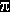. The mass m is defined as:where:

•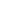t = operation time (one operation, essentially one quantum change)
• M = perceived mass
•Ai = action in to (created in) volumetric region occupied by the mass, i.e., action created in that volumetric region from fusing virtual photons into quanta (photons)
•Ao = action lost from volumetric region occupied by mass, i.e., action lost from that volumetric region from fissioning of quanta (photons) into virtual quanta (virtual photons)
•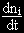= number of k-sized bundles of virtual photons per second fused into quanta, in the mass volumetric region.
•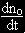= number of quanta per second fissioned into virtual photons, in the mass volumetric region
• k = Planck's constant divided by 4As can be seen, we are simply saying that, in a volumetric region occupied by a mass, a special fissioning and fusioning of action quanta are occuring. The mass is simply the activity rate of that fission/fusion activity, multiplied by some constant of proportionality to make the constants turn out right.

Mass is assumed to be a high condensation/concentration of energy in that local volume.

The fundamental notion is that, to produce energy, a quantum must be fissioned; i.e., one must split the quantum (E)(t) to produce a piece of energy (E). This (E)/c2 gives the degree of condensation/concentration, and hence the "mass" of this occurence, but that mass is linked to the (t) left from conversion of the energy, so that no mass M but masstime MT exists momentarily. In the next instance, the mass M of this masstime "reconverts" to (E) via E=mc2, and this (E) recombines with the (t) portion of the MT, to form a photon which moves off, leaving behind a 3-space M which is not changing.

Note that mass itself does not exist in time, but only in 3-space. The emission of the photon "tears off" the time-tail of the 4-space MT, leaving behind only 3-space M. This is why time is not an observable in quantum mechanics; every mass is continually absorbing and emitting photons at an incredible rate. The emission of every photon converts 4-space (L3T) to 3-space (L3). The next absorption of a photon changes the Mass into Masstime momentarily. Thus a mass is continually "jumping" through time in little jumps, which are discretized but not quantized. Indeed, any finite mass is doing this in a fantastic stream of photon absoption/emission interactions, particularly at the virtual photon level. But every physical instrument, being made of mass, and being in an incredible stream of this action fission/fusion exchange with the surrounding vacuum, imposes a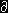/t operator upon (L3T) spacetime in its measurement process, leaving (L3) in the remaining "observation" or "measurement". This is why "all measurement/observation is spatial". It also accounts for the Heisenberg uncertainty principle -- which is a detection/measurement principle, not an "existing as" principle.

The "rest mass" (motionless) mass of a mass identically is simply the intensity of this interaction with virtual photons, i.e. with of the virtual photon flux of the vacuum, of a particular region of "curved spacetime" that is "occupied" by that mass. When a mass (i.e., curved ST volumetric region) is in motion with respect to the observer, the mass meets a greater flux of virtual photons along its line of travel; hence this activity rate increases with respect to that direction, hence the "mass" -- which is just this very activity rate -- is greater in that direction. This is the mechanism for the so-called "relativistic" increase of mass with velocity. Note that, at right angles to the direction of motion, the mass M of the object remains its rest mass.

Note also that, by quantum field theory, all EM and mechanical forces are due to the exchange of virtual photons. It follows that any additional force brought to bear upon a mass, or exerted by it upon another mass, must be due to a change in the local vacuum flux, and hence in the activity rate comprising the mass. In short, it alters the local energy density and virtual photon flux of vacuum, thereby curving spacetime. This "curved spacetime" then interacts upon the recipient mass. Hence Wheeler's statement of general relativity that "mass tells spacetime how to curve, and (curved) spacetime tells mass how to move".

Note also that the same fission/fusion that establishes the mass in a volumetric region, also establishes the rate at which Delta t's are produced and reabsorbed in photons. In short, the same process that creates mass, creates the rate of flow of time for that mass.

Since the exterior of an atom is the electron shells, the interactions of the gravitons of the ambient vacuum potential split into separate photon and antiphoton interactions. The photons interact with the orbital electrons, are absorbed then re-emitted. But the orbital motion means that re-emission may be in a different direction. So the emitted photons are "scattered" with respect to the "ordering" with which they entered to interact with the atom. This creates the "entropy" of that demon, thermodynamics. On the other hand, in the nucleus perfect reordering (negentropy) always occurs (except for PCMs), but we just call that "Newton's third law" and ignore it.

The point is, entropy can be directly engineered and reversed or beaten. E.g., when one performs work, one only "scatters" the energy (photons) as heat, or changes the form of the energy. No energy is lost whatsoever. Let us examine a purely heat reaction, where all the energy (order) is scattered as scattered photons. Imagine that the scatterer is a heater wire. Simply surround the scatterer by a phase conjugate mirror material, in the form of a closed cylinder (with a negligeable little hole in the end through which the heater wire is inserted) with an internal reflectivity coefficient of 0.5. Now one half of the emitted photons from the heater wire in the cylinder will be returned precisely to the origination point, so one "recovers" one half the energy from each "snapshot" examined. This gives a series of the energy in the heater wire being 1 + 1/2 + 1/4 + .... This well-known series is convergent upon the sum of 2.0. So one eventually has twice the energy inside the cylinder as one is putting into the wire. Half this energy is being lost through the walls of the cylinder and radiated away, so one sees as much radiated away as one sees himself feeding into the heater. Hey, no overunity here! Now simply use a material with a cylinder material with a phase conjugate coefficient of reflectivity slightly greater than 0.5. The new series is divergent; i.e., energy inside the cylinder increase without limit, as does the amount of heat emitted by the outer surface of the cylinder. Hey! After a bit, you are putting in a certain, steady amount of power, and the power radiated from the outer cylinder is continually increasing. Now you have an overunity machine. No violation of the conservation of energy law, of the laws of physics, or the laws of thermodynamics is occuring.

The point is, energy which has been "used" in this case is just scattered energy. It can be continually recovered and "reused" again and again, by a simple retroreflective operation. This is one way you really can make a true "Maxwell's demon".

At any rate, eventually, I simply treated the EM energy representing the mass as a special kind of scalar electrostatic potential, of powerful magnitude. In so doing, it can be mathematically decomposed into bidirectional EM wavepairs, according to Whittaker. [Whittaker wrote the sum set of waves; Ziolkowski added the product set as well, which means not only frequencies but modulations (products of frequencies).] In theory then, it is possible to take a specific mass, and it will have a specific patterning of these Whittaker/Ziolkowski waves. In theory, arrays of dipoles, augmented by nuclear magnetic detectors, could be developed to detect and portray these frequencies and their products, say, for a specific element. One could then take a similar set, say, for an isomer of a radioactive nucleus [that] one desires to transform into something harmless. Then one could irradiate the harmful radioactive element with a "delta" potential whose internal W-Z composition was precisely tailored to accomplish the change along the isomer chain that is desired. In that manner, after some irradiation, the harmful nuclei could be transformed into the new, desired, less harmful nuclei. In this way, direct transmutation of matter could be accomplished.

Of course, eventually, you could also make gold or platinum from baser elements if desired. Or one could make some of the highly valuable and useful radioactive isotopes that are needed in industry and medicine.

The point is, by deliberately structuring the scalar potential, one can directly engineer (1) the nucleus, (2) the type element or mass, (3) gravity and antigravity, (4) transmutation, (5) the rate of passage of time, including local time reversal, and (6) overunity energy device of great power. Note that local time reversal of an object or mass is not time travel. One does not keep the local identity in its "present", while time reversing all the rest of the universe! That is what would have to be done to get the science fiction notion of "travel to the past". We are not suggesting that! But one local entity -- including a human cell or an entire body -- can in fact be time-reversed to a previous state, and the proof is already in the open literature. E.g., Becker and others have demonstrated the ability to differentiate and dedifferentiate cells. Note that if you simply dedifferentiate a diseased cell, such as one infected by AIDS, back to a former state when it was healthy, all the DNA etc. are altered also. In this way, infectious diseases -- such as cancer, AIDS, the Ebola virus, etc. -- could be readily cured.

This also has been experimentally proven in the late 1960s and 1970s by the Priore team in France, under rigorous scientific protocols, and reported in the open scientific literature. A complete digest of the Priore affair and the supression of the magnificient new medical approach is given by Christopher Bird, "The Case of Antoine Priore and His Therapeutic Machine: A Scandal in the Politics of Science", Appendix I in T.E. Bearden, AIDS: Biological Warfare, Tesla Book Co., P.O. Box 121873, Chula Vista, California, 91912. An updated version is Christopher Bird, "The Case of Antoine Priore and His Therapeutic Machine: A Scandal in the Politics of Science", Explore!, 5(5-6), 1994, p.97-110. A complete, detailed exposition of the technical mechanism for the Priore curative therapy is given by Bearden, T.E. (1995) "Vacuum engines and Priore's methodology: The true Science of energy-medicine". Part I of II. Explore!, 6(1), p.66-76; Part II of II, Explore!, 6(2), 1995, p.50-62.

Again, thanks for including my material on the internet. Good luck to you, and we will have some disks to you shortly.

Sincerely,

Tom Bearden, June 1, 1995